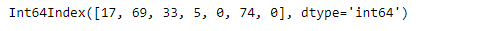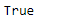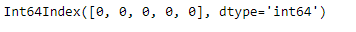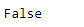# Python | Pandas Index.any()

• Last Updated : 16 Dec, 2018

Python is a great language for doing data analysis, primarily because of the fantastic ecosystem of data-centric python packages. Pandas is one of those packages and makes importing and analyzing data much easier.

Pandas` Index.any()` function checks if any of the elements in the index are true or not. It returns one single boolean value if axis is not specified. It returns true if any one value in the index is true. It returns false if none of the values in the index is true.

Note : It treats 0 as false value.

Syntax: Index.any(*args, **kwargs)

Parameters :
*args : These parameters will be passed to `numpy.any`
**kwargs : These parameters will be passed to `numpy.any`

Returns : any : bool or array_like (if axis is specified)
A single element array_like may be converted to bool.

Example #1: Use `Index.any()` function to check if all values are true in the index.

 `# importing pandas as pd``import` `pandas as pd`` ` `# Creating the Index``df ``=` `pd.Index([``17``, ``69``, ``33``, ``5``, ``0``, ``74``, ``0``])`` ` `# Print the dataframe``df`

Output :Let’s check if any of the values in the index is true or it is having all the false value.

 `# to check if there is any false ``# value present in the index``df.``any``()`

Output :As we can see in the output, the function has returned true indicating that there is at least one true value present in the Index.

Example #2: Use `Index.any()` function to check if any value in the index is true or not.

 `# importing pandas as pd``import` `pandas as pd`` ` `# Creating the Index``df ``=` `pd.Index([``0``, ``0``, ``0``, ``0``, ``0``])`` ` `# Print the dataframe``df`

Output :Let’s check if any of the values in the index is true or it is having all the false value.

 `# to check if there is any false``#  value present in the index``df.``any``()`

Output :My Personal Notes arrow_drop_up